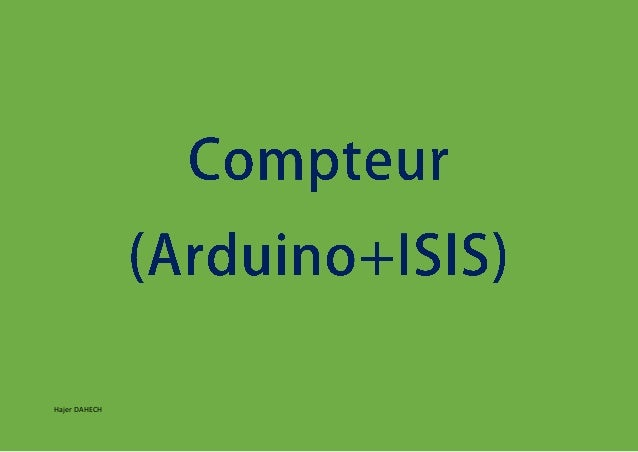Successfully reported this slideshow.Upcoming SlideShare
×

# Compteur ARDUINO

3,519 views

Published on

CODE & VIDEO TUTORIAL
https://hajereducation.tn/comment-creer-un-compteur-de-0-a-99-avec-arduino-proteus7_isis/
SYSTÈME EMBARQUE
https://hajereducation.tn/category/embedded-system-projects/

Published in: Software
• Full Name
Comment goes here.

Are you sure you want to Yes NoAre you sure you want to  Yes  No

Are you sure you want to  Yes  No

Are you sure you want to  Yes  No

Are you sure you want to  Yes  No

Are you sure you want to  Yes  No

### Compteur ARDUINO

1. 1. Hajer DAHECH
2. 2. Hajer DAHECH const int bit_A = 2; const int bit_B = 3; const int bit_C = 4; const int bit_D = 5; const int bit_A1 = 8; const int bit_B1 = 9; const int bit_C1 = 10; const int bit_D1= 11; Code Arduino
3. 3. Hajer DAHECH void setup() { //on met les broches en sorties pinMode(bit_A, OUTPUT); pinMode(bit_B, OUTPUT); pinMode(bit_C, OUTPUT); pinMode(bit_D, OUTPUT); pinMode(bit_A1, OUTPUT); pinMode(bit_B1, OUTPUT); pinMode(bit_C1, OUTPUT); pinMode(bit_D1, OUTPUT);
4. 4. Hajer DAHECH //on commence par écrire le chiffre 0, donc toutes les sorties à l'état bas digitalWrite(bit_A, LOW); digitalWrite(bit_B, LOW); digitalWrite(bit_C, LOW); digitalWrite(bit_D, LOW); digitalWrite(bit_A1, LOW); digitalWrite(bit_B1, LOW); digitalWrite(bit_C1, LOW); digitalWrite(bit_D1, LOW);
5. 5. Hajer DAHECH } //fonction écrivant sur un afficheur U1 void afficher(char chiffre) { switch(chiffre) { case 0 : digitalWrite(bit_A, LOW); digitalWrite(bit_B, LOW);
6. 6. Hajer DAHECH digitalWrite(bit_C, LOW); digitalWrite(bit_D, LOW); break; case 1 : digitalWrite(bit_A, HIGH); digitalWrite(bit_B, LOW); digitalWrite(bit_C, LOW); digitalWrite(bit_D, LOW); break; case 2 : digitalWrite(bit_A, LOW);
7. 7. Hajer DAHECH digitalWrite(bit_B, HIGH); digitalWrite(bit_C, LOW); digitalWrite(bit_D, LOW); break; case 3 : digitalWrite(bit_A, HIGH); digitalWrite(bit_B, HIGH); digitalWrite(bit_C, LOW); digitalWrite(bit_D, LOW); break; case 4 :
8. 8. Hajer DAHECH digitalWrite(bit_A, LOW); digitalWrite(bit_B, LOW); digitalWrite(bit_C, HIGH); digitalWrite(bit_D, LOW); break; case 5 : digitalWrite(bit_A, HIGH); digitalWrite(bit_B, LOW); digitalWrite(bit_C, HIGH); digitalWrite(bit_D, LOW); break;
9. 9. Hajer DAHECH case 6 : digitalWrite(bit_A, LOW); digitalWrite(bit_B, HIGH); digitalWrite(bit_C, HIGH); digitalWrite(bit_D, LOW); break; case 7 : digitalWrite(bit_A, HIGH); digitalWrite(bit_B, HIGH); digitalWrite(bit_C, HIGH); digitalWrite(bit_D, LOW);
10. 10. Hajer DAHECH break; case 8 : digitalWrite(bit_A, LOW); digitalWrite(bit_B, LOW); digitalWrite(bit_C, LOW); digitalWrite(bit_D, HIGH); break; case 9 : digitalWrite(bit_A, HIGH); digitalWrite(bit_B, LOW); digitalWrite(bit_C, LOW);
11. 11. Hajer DAHECH digitalWrite(bit_D, HIGH); break; } } //fonction écrivant sur afficheur U2 void afficher1(char chiffre) { switch(chiffre) { case 0 : digitalWrite(bit_A1, LOW);
12. 12. Hajer DAHECH digitalWrite(bit_B1, LOW); digitalWrite(bit_C1, LOW); digitalWrite(bit_D1, LOW); break; case 1 : digitalWrite(bit_A1, HIGH); digitalWrite(bit_B1, LOW); digitalWrite(bit_C1, LOW); digitalWrite(bit_D1, LOW); break; case 2 :
13. 13. Hajer DAHECH digitalWrite(bit_A1, LOW); digitalWrite(bit_B1, HIGH); digitalWrite(bit_C1, LOW); digitalWrite(bit_D1, LOW); break; case 3 : digitalWrite(bit_A1, HIGH); digitalWrite(bit_B1, HIGH); digitalWrite(bit_C1, LOW); digitalWrite(bit_D1, LOW); break;
14. 14. Hajer DAHECH case 4 : digitalWrite(bit_A1, LOW); digitalWrite(bit_B1, LOW); digitalWrite(bit_C1, HIGH); digitalWrite(bit_D1, LOW); break; case 5 : digitalWrite(bit_A1, HIGH); digitalWrite(bit_B1, LOW); digitalWrite(bit_C1, HIGH); digitalWrite(bit_D1, LOW);
15. 15. Hajer DAHECH break; case 6 : digitalWrite(bit_A1, LOW); digitalWrite(bit_B1, HIGH); digitalWrite(bit_C1, HIGH); digitalWrite(bit_D1, LOW); break; case 7 : digitalWrite(bit_A1, HIGH); digitalWrite(bit_B1, HIGH); digitalWrite(bit_C1, HIGH);
16. 16. Hajer DAHECH digitalWrite(bit_D1, LOW); break; case 8 : digitalWrite(bit_A1, LOW); digitalWrite(bit_B1, LOW); digitalWrite(bit_C1, LOW); digitalWrite(bit_D1, HIGH); break; case 9 : digitalWrite(bit_A1, HIGH); digitalWrite(bit_B1, LOW);
17. 17. Hajer DAHECH digitalWrite(bit_C1, LOW); digitalWrite(bit_D1, HIGH); break; } } void loop() { char i=0; //variable "compteur U1" char j=0; //variable "compteur U2" for(i=0; i<10; i++) {
18. 18. Hajer DAHECH for(j=0; j<10; j++){ afficher(j); //appel fonction d'affichage U1 afficher1(i); // appel fonction d'affichage U2 delay(1000); //on attend 1 seconde } delay(1000); //on attend 1 seconde } }
19. 19. Hajer DAHECH ISIS